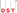# Dividends, earnings, and cash flow discount models

•DST Systems, Inc.
• Accounting Method
• Accounting Method

Financial theory posits that the value of an investment can be seen as the sum of the future cash flows the investment is expected to produce. Through that lens, a stock investment can be looked at as some combination of earnings, cash flow, or dividend streams, plus the potential increase in share value that could be realized by the eventual liquidation of the investment.

At the base of the valuation process is the concept of financial discounting—the idea that a payment deferred into the future should be worth more than a payment made today in order to compensate the recipient for the costs and risks inherent in waiting. Thus, a series of payments into the future is not merely the sum of those payments, but a transformation of that series into a single number that represents the present-day equivalent value of the cash flow. That value is known generically as present value (PV). A related figure, net present value (NPV), factors in direct costs incurred by the investor to realize the cash flows, such as the foreseeable taxes due on dividend payments or additional capital inputs that might be required by future calls.

The baseline cost in discounting is typically an opportunity cost; specifically, it is the income the investor foregoes by not putting the same value into a risk-free investment. Admittedly, a truly risk-free investment exists only in theory. However, in practice, the prevailing interest rate on short-term notes issued by the US Treasury is often used as an approximation since these securities are widely believed to have negligible credit and redemption risks. In mature markets other than the United States, investors typically use that market's short-term sovereign debt as the applicable risk-free benchmark. In markets with unstable sovereign debt, there may be no functional surrogate rate in the local currency that could be called risk free, making this type of analysis more complex.

The risk of nonpayment is the other major factor in determining a discount rate and it tends to be more difficult to assess. Companies with historically stable earnings, dividends, or free cash flow combined with stable outlooks for future performance may be seen as being relatively low risk in those dimensions. They tend to require a relatively small excess charge over the risk-free base rate used in discounting models, so they are said to have a relatively small risk premium. However, as the net variability in any expected performance dimension increases, so does the investor's desired risk premium, increasing the discount rate in that dimension. The larger the discount rate is, the bigger the reduction from future value to present value will be.

## Practical applications of theory

### The dividend discount model

The dividend discount model (DDM) is a method for assessing the present value of a stock based on its dividend rate.

If the company currently pays a dividend and you assume that the dividend will remain constant indefinitely, then the present value of the dividend would simply be dividend dollar amount divided by the desired discount rate. For example, a stock that is expected to pay a \$2 annual dividend in a market environment that supports a 7% discount rate would have a present value for its dividend stream of \$28.57.

If a company were expected to grow its dividend by a constant rate indefinitely, then the present value would be the current dividend amount divided by the difference between the discount rate and the expected growth rate (this only works arithmetically when the expected growth rate is less than the dividend rate). Given the baseline values indicated previously, the present value of the dividend stream in the second scenario would be \$50 (\$2/(7% − 3%)).

Keep in mind that dividend scenarios other than indefinite stability or indefinite growth at a steady rate would require more complex computations.

### The earnings discount model

Traditional dividend discount calculations do not consider any broader views of company performance or management policy in their evaluation. The earnings discount model addresses that by factoring in payout ratio, or the proportion of earnings devoted to dividend payments. Take the payout ratio (the current dividend divided by the current earnings per share) and divide that by the difference between the investor's discount rate and the dividend growth rate. The result is the earnings discount model's P/E, which can then be compared to the market's P/E.

### The discounted cash flow model

Discounted cash flow (DCF) valuation is based entirely on the internal dynamics of the company. It assesses every element of free cash flow the company is expected to produce, and then discounts that flow using the company's own weighted average cost of capital. The value that DCF valuation produces can be compared to the current company market capitalization or to break-up value.

Valuations generated by discount models can be useful comparative indicators but there are no absolute action rules. For example, a dividend discount valuation that is higher than the current stock price may point to a buying opportunity, but it may also signal that the market does not agree with expected dividend growth assessment or it feels the discount rate may be too high. Similarly, an earnings discount model P/E that's higher than the market P/E may signal a buying opportunity, or it may indicate that the market's assessment of company earnings quality is low, so the market puts little actionable faith in the higher reported number. And a discounted cash flow valuation may point to hidden value in future performance potential, or it may signal overly optimistic assumptions about that potential.